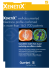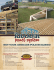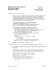# Examination Cover Sheet Princeton University Undergraduate Honor Committee January 18, 2008

## Transcription

Examination Cover Sheet Princeton University Undergraduate Honor Committee January 18, 2008
```Examination Cover Sheet
January 18, 2008
Course Name & Number: PHY105
Professors: Chris Herzog, Lyman Page, Jason Petta
Date: January 19, 2008
Time: 9:00 AM
This examination is administered under the Princeton University Honor Code. Students
should sit one seat apart from each other, if possible, and refrain from talking to other
students during the exam. All suspected violations of the Honor Code must be reported to
the Honor Committee Chair at [email protected]
The checked items are permitted for use on this examination. Any item that is not
checked may not be used and should not be in your working space. Assume items not on
this list are not allowed for use on this examination. Please place items you will not need
out of view in your bag or under your working space at this time. University policy does not
allow the use of electronic devices such as cell phones, PDAs, laptops, MP3 players, iPods,
etc. during examinations. Students may not wear headphones during an examination.
2 Course textbooks: No
2 Course Notes: No
2 Other books/printed materials: No
x Formula Sheet: Yes, but only the one provided
2 Comments on use of printed aids: None
Students may only leave the examination room for a very brief period without the explicit
permission of the instructor. The exam may not be taken outside of the examination room.
This exam is a timed examination. You will have
3 hours
0 minutes
to complete this exam.
During the examination, the Professor will be outside the door.
On the cover of your first booklet, write and sign the Honor Code pledge:
“I pledge my honor that I have not violated the Honor Code during this examination”
1
This exam consists of seven problems. When we begin, check to see that this copy of
the exam has all seven. Use the same exam booklet for all problems, continuing to another
booklet if necessary. Print your name on each booklet as you start it.
USE OF CALCULATORS IS NOT PERMITTED!!
At the end of the exam, indicate clearly on the cover of your first exam booklet how
many booklets you used.
Some useful test-taking hints:
• You may not be able to complete every problem. Keep moving – do what you know
first.
• Use symbols rather than numbers wherever possible and CHECK UNITS.
• Whenever possible, check whether an answer or intermediate result makes sense before
moving on.
• There is a list of formulas on the last page. You can tear it off. Use it as a reminder
of details. Don’t try to do problems by searching through the sheet!
• If you get stuck on an early part of a problem, check the later parts — some may be
independent and doable.
• If you get stuck on an early part of a problem, and a later part depends on it, clearly
define a symbol for the unknown answer and use it in later parts. Note: this is an act
of desperation – we often give multiple parts to guide you through a problem.
2
1. Double Atwood System [20 pts]. A double Atwood system consists of masses M1
and M2 , which are connected by massless strings, as shown below. Assume that the pulleys,
P1 and P2 , are massless and frictionless, and that the string is massless. The wide grey bands
indicate the ceiling and floor and are fixed.
a) [10 pts] Call a1 the acceleration of M1 and a2 the acceleration of M2 . What is the
relation between a1 and a2 ? Clearly label your coordinate system and show your work.
b) [10 pts] What is a1 in terms of M1 , M2 , and g?
2. Firing Block [20 pts]. A block of wood of mass mw and dimensions 20 cm length by
8 cm width by 2 cm thick has a massless rod through its center of mass and perpendicular to
one side. The rod is set atop some supports so that the two large dimensions of the block are
in a plane parallel to the ground as shown. A gun is fired from below the block so that the
bullet of initial velocity vb and mass mb strikes the block roughly 8 cm out from the center
along its length but centered in width. The bullet is embedded in the wood. The block rises
and spins as a result of the off-center shot. In the limit of mb << mw , how high does its
center of mass rise? You may neglect the thickness (4 cm) when computing the height.
3
3. Teeter Toy [30 pts]. A teeter toy, shown below, consists of two weights of mass m,
which hang from a peg attached to two arms at a fixed angle α. We wish to determine the
stability of the teeter toy when the peg is rotated from the vertical by an angle θ. You may
consider the arms and peg as massless.
θ
α
α
a) [10 pts] What is the potential energy, U(θ)? For simplicity, take the zero of the
gravitational potential energy to coincide with the pivot point.(Hints: Use the identity
for cos(α ± β) from the formula sheet to simplify your expression, use only the angles
and lengths in the diagram, and notice that the weights must move on a circle.)
b) [5 pts] For what value(s) of θ is the system in equilibrium?
c) [5 pts] What is the condition on L, l, and α for the equilibrium to be stable?
d) [10 pts] In general, the potential energy, U, and the kinetic energy, K, of a harmonic
oscillator can be written as:
1
U = Aq 2 + const.
(1)
2
and
1
K = B q˙2
(2)
2
where A and B are constants. How is the oscillation frequency related to A and B?
What is the frequency of small oscillations of the teeter toy?
4
4. Stepin’ Sphere [20 pts]. A sphere of radius R and mass m rolls without slipping
toward a vertical step of height h where h < R. We want to find the initial rolling velocity,
v0 , at which the ball just makes it over the step. In the collision with the step, you may
assume that the ball briefly “sticks” to the edge until its center of mass is directly over the
edge. The moment of inertia of a sphere is 2mR2 /5 about its center of mass.
a) [5 pts] What is the angular momentum of the ball with respect to the edge of the step
before the collision?
b) [15 pts] What is the initial velocity required so that the ball just climbs over the step?
5. Strange Circles [20 pts]. In polar coordinates (r, θ), a particle of mass m moves
in a circle r = 2R cos θ (−π/2 ≤ θ ≤ π/2) under the influence of a central force potential
U(r) = −K/r α . Notice that this orbit passes through the origin. Though the orbit is
unstable, this fact does not concern us. There are no relativistic effects.
a) [10 pts] What is the total energy, E, of the orbit?
b) [5 pts] What must α be?
c) [5 pts] What is its angular momentum, l, in terms of m, R, & K?
5
6. Bottle Tunes [20 pts]. A bottle of total volume V has a narrow cylindrical neck
at the top of cross sectional area A. The bottle sits at equilibrium in a room at a pressure
P0 and fixed temperature. The bottle is sealed by placing a light cylinder of mass m in the
neck. The cylinder can slide frictionlessly up and down inside the neck but does not let gas
pass from one side to the other.
a) [10 pts] After a suitable time, a new equilibrium is reached with the cylinder inside the
neck but a distance h0 from the top and the gas in the bottle at the same temperature
as the room. What is h0 in terms of V , A, P0 , m, and g? You may assume that
mg << AP0 .
b) [10 pts] If the cylinder is displaced a small distance away from equilibrium, x, it
oscillates with frequency ω. Assuming the compression of the gas in the bottle is
adiabatic (unlike the isothermal compression on PS10), what is ω? You may assume
that Ax << V .
[No points] This oscillation is meant to be a toy model of the effect of blowing on a soda
bottle where the cylinder of mass m is the air mass inside the neck of the bottle. Given
A = π/4 cm2 , V = 5.6L, m = 10−3 g, and a pressure of about one atmosphere, P0 = 106
dyn cm−2 . In the solutions we will post the frequency of oscillation.
h0
m
P
6
P0
7. The Pole in the Barn [20 pts]. A farmer owns a barn with electric doors on opposite
ends that she can close extremely rapidly and simultaneously. The length of the barn is 4l0 /5
as shown in the figure. A pole vaulter has a pole that has a length l0 as measured at rest by
the farmer.
a) [5 pts] The farmer says to the pole vaulter “if you run fast enough, your pole will
contract and just as you pass through the barn I’ll be able to close both doors (and
then quickly open them) and have you inside for an instant.” How fast does the pole
vaulter have to run to fit inside the barn and have the doors close?
The pole vaulter says “no way! according to me the barn will be Lorentz contracted
and I’ll smash the doors when you close them for that instant.” Assume that the
farmer and pole vaulter sync up their observations when the back of the pole is aligned
with the left hand (“A”) door.
Call the pole vaulter coordinates x0 and t0 and express all times and distances in terms
of l0 and c. For the pole vaulter:
b) [5 pts] When does the “B” (right hand) door close and then quickly open?
c) [5 pts] How long does the pole vaulter think he is in the barn?
d) [5 pts] Is the pole vaulter correct? Does any wood get splintered? Briefly describe the
Barn
v
Pole
A
B
⁄ l
l
7
Physics 105 Formula Sheet
µ=
Mm
,
M +m
Ueff =
2
ac =
v
,
r
r=
l2
+U(r),
2µr 2
r0
,
1 − cos θ
F=−
GMm
ˆr,
r2
r0 ≡ l2 /µC,
≡
U =−
s
GMm
,
r
T2 =
π 2 µA3
2C
2El2
,
µC 2
C ≡ GMm
2πf = ω,
T = 1/f
1+
A = −C/E
x¨ + γ x˙ +
x¨ + ω02 x = 0,
ω02 = k/m,
eiφ = cos φ + i sin φ,
ω02 x
=0
F
or =
m
x = A cos(ω0 t + φ),
z = x + iy = reiφ ,
y(x, t) = f (x ∓ vt),
r 2 = zz ∗ ,
y(x, t) = A cos(kx ∓ ωt)
v=
tan φ =
ω
λ
= ,
T
k
Imz
Rez
λ=
2π
k
0
fsound
= f0 (vs ± vrec )/(vs ∓ vsrc )
sin α + sin β = 2 sin
α−β
α+β
cos
,
2
2
cos α + cos β = 2 cos
sin(α ± β) = sin α cos β ± cos α sin β,
α+β
α−β
cos
2
2
cos(α ± β) = cos α cos β ∓ sin α sin β
Lorentz transform : x0 = γ(x − vt), y 0 = y, z 0 = z, t0 = γ(t − xv/c2 )
x = γ(x0 + vt0 ), y = y 0, z = z 0 , t = γ(t0 + x0 v/c2 )
β = v/c,
p
γ = 1/ 1 − β 2 ,
E = γm0 c2 ,
p = γm0 v,
ux = (u0x + v)/(1 + vu0x /c2 ),
E 2 = p2 c2 + m20 c4 ,
uy = u0y /γ(1 + vu0x /c2 )
E = pc (f or m0 = 0)
For a planar object rotationally symmetric about z:
 R 2

ρy dV R 0
0
↔

0
ρx2 dV R
0
I=
2
2
0
0
ρ(x + y ) dV
8
```

### Exam 3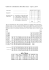### 3w 3 t5+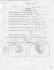### 2 for 1 Dinner Menu - Club Regent Casino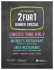### Examination Cover Sheet Princeton University Undergraduate Honor Committee January 17, 2007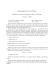### Byzantine ARt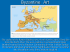### STEP OUT FOR A COLORFUL AUTUMN TREK!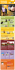### HERE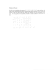### Questions by Maryland`s Phil Durkos, Brad Houston, Eric Newman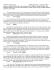### STL`s favorite backyard games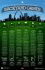### 4 P XENETIX P07021 190207.qxd